# Minimally Sufficient PandasIn this article, I will offer an opinionated perspective on how to best use the Pandas library for data analysis. My objective is to argue that only a small subset of the library is sufficient to complete nearly all of the data analysis tasks that one will encounter.

This minimally sufficient subset of the library will benefit both beginners and professionals using Pandas. Not everyone will agree with the suggestions I lay forward, but they are how I teach and how I use the library myself. If you disagree or have any of your own suggestions, please leave them in the comments below.

• Know why limiting Pandas to a small subset will keep your focus on the actual data analysis and not on the syntax
• Have specific guidelines for taking a single approach to completing a variety of common data analysis tasks with Pandas

# Pandas is Powerful but Difficult to use

Pandas is the most popular Python library for doing data analysis. While it does offer quite a lot of functionality, it is also regarded as a fairly difficult library to learn well. Some reasons for this include:

• There are often multiple ways to complete common tasks
• There are over 240 DataFrame attributes and methods
• There are several methods that are aliases (reference the same exact underlying code) of each other
• There are several methods that have nearly identical functionality
• There are many tutorials written by different people that show different ways to do the same thing
• There is no official document with guidelines on how to idiomatically complete common tasks
• The official documentation, itself contains non-idiomatic code

# What is Minimally Sufficient Pandas?

The whole point of a data analysis library should be to provide you with the tools so that you can focus on the data analysis. While Pandas does provide you with the right tools, it doesn’t do so in a way that allows you to focus on the analysis. Instead, users are forced to tread through the complex and overabundant syntax.

I endorse the following as my definition for Minimally Sufficient Pandas.

• It is a small subset of the library that is sufficient to accomplish nearly everything that it has to offer.
• It allows you to focus on doing data analysis and not the syntax

With this minimally sufficient subset of Pandas:

• Your code will be simple, explicit, straightforward, and boring
• You will choose one obvious way to accomplish a task
• You will use this obvious way every single time
• You won’t have to retain as many commands in working memory
• Your code will be easier to understand by others and by you

Pandas often gives its users multiple approaches to complete the same task. This means that your approach may use different syntax than someone else’s. This can occur even with the most rudimentary tasks such as selecting a single column of data. Using multiple different syntaxes might not lead to many issues during a single analysis done by a single person. However, it can cause havoc when a team of people are working through a long analysis using all different approaches to Pandas.

By not having a standard approach to common tasks, a larger cognitive load is placed on the developer, who must remember all the slight differences to each approach. Having more than a single way to complete each common task is asking to introduce errors and inefficiencies.

## Avalanche of Stack Overflow Answers

It is not uncommon to search for Pandas answers on Stack Overflow only to be met with several competing and varied results for common tasks. This particular question about renaming columns in a DataFrame has 28 answers. Treading through this deluge of information makes it difficult for those wanting to know the one idiomatic way to complete a task that they can commit to memory.

## No Tricks

Eliminating much of the library will come with some (good) limitations. Knowing many obscure Pandas tricks might impress your friends, but it doesn’t usually lead to good code. It can lead to long lines of code that are difficult to understand and may be harder to debug.

# Specific Pandas Examples

We will now cover a series of specific examples within Pandas where multiple approaches exist to complete a task. I will compare and contrast the different approaches and give guidance on which one I prefer. Listed below are the topics I cover.

• Selecting a single column of data
• The deprecated `ix` indexer
• Selection with `at` and `iat`
• `read_csv` vs `read_table` duplication
• `isna` vs `isnull` and `notna` vs `notnull`
• Arithmetic and Comparison Operators and their Corresponding Methods
• Builtin Python functions vs Pandas methods with the same name
• Standardizing `groupby` aggregation
• Handling a MultiIndex
• The similarity between `groupby`, `pivot_table` and `crosstab`
• `pivot` vs `pivot_table`
• The similarity between `melt` and `stack`
• The similarity between `pivot` and `unstack`

# Minimally Sufficient Guiding Principle

The concrete examples were all derived by the following principle:

If a method does not provide any additional functionality over another method (i.e. its functionality is a subset of another) then it shouldn’t be used. Methods should only be considered if they have some additional, unique functionality.

# Selecting a Single Column of Data

Selecting a single column of data from a Pandas DataFrame is just about the simplest task you can do and unfortunately, it is here where we first encounter the multiple-choice option that Pandas presents to its users.

You may select a single column as a Series with either the brackets or dot notation. Let’s read in a small, trivial DataFrame and select a column using both methods.

``````>>> import pandas as pd
>>> df
``````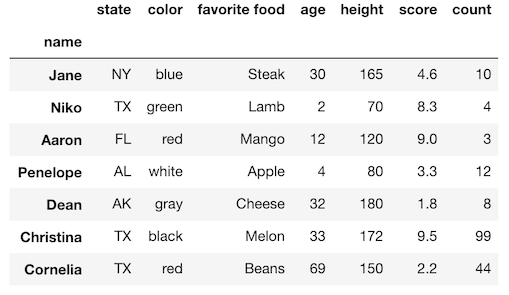## Selection with the brackets

Placing a column name in the brackets appended to a DataFrame selects a single column of a DataFrame as a Series.

``````>>> df['state']
name
Jane         NY
Niko         TX
Aaron        FL
Penelope     AL
Dean         AK
Christina    TX
Cornelia     TX
Name: state, dtype: object
``````

## Selection with dot notation

Alternatively, you may select a single column with dot notation. Simply, place the name of the column after the dot operator. The output is the exact same as above.

``````>>> df.state
``````

## Issues with the dot notation

There are three issues with using dot notation. It doesn’t work in the following situations:

• When there are spaces in the column name
• When the column name is the same as a DataFrame method
• When the column name is a variable

## There are spaces in the column name

If the desired column name has spaces in it, you won’t be able to select it with the dot notation. Python uses spaces to separate names and operators and hence will not treat a column name with a space as correct syntax. Let’s create this error.

``````df.favorite food
``````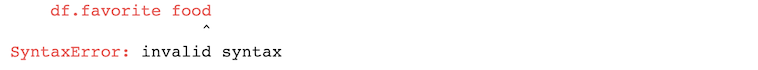You can only use the brackets to select columns with spaces.

``````df['favorite food']
``````

## The column name is the same as a DataFrame method

When a column name and a DataFrame method collide, Pandas will always reference the method and not the column name. For instance, the column name`count` is a method and will be referenced when using dot notation. This actually doesn’t produce an error as Python allows you to reference methods without calling them. Let’s reference this method now.

``````df.count
``````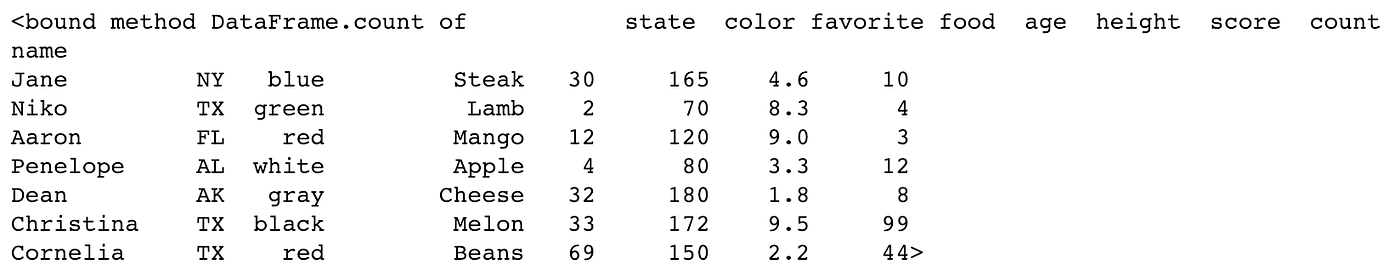The output is going to be very confusing if you haven’t encountered it before. Notice at the top it states ‘bound method DataFrame.count of’. Python is telling us that this is a method of some DataFrame object. Instead of using the method name, it outputs its official string representation. Many people believe that they’ve produced some kind of analysis with this result. This isn’t true and almost nothing has happened. A reference to the method that outputs the object’s representation has been produced. That is all.

Regardless, it’s clear that using dot notation did not select a single column of the DataFrame as a Series. Again, you must use the brackets when selecting a column with the same name as a DataFrame method.

``````df['count']
``````

## The column name is a variable

Let’s say you are using a variable to hold a reference to the column name you would like to select. In this case, the only possibility again is to use the brackets. Below is a simple example where we assign the value of a column name to a variable and then pass this variable to the brackets.

``````>>> col = 'height'
>>> df[col]
``````

## The brackets are a superset of dot notation

The brackets are a strict superset of the dot notation in terms of functionality for selecting a single column. There are three cases which are not handled by the dot notation.

## Lots of Pandas is written with the dot notation. Why?

Many tutorials make use of the dot notation to select a single column of data. Why is this done when the brackets seem to be clearly superior? It might be because the official documentation contains plenty of examples that use it. It also uses three fewer characters which entice the very laziest amongst us.

## Guidance: Use the brackets for selecting a column of data

The dot notation provides no additional functionality over the brackets and does not work in all situations. Therefore, I never use it. Its single advantage is three fewer keystrokes.

I suggest using only the brackets for selecting a single column of data. Having just a single approach to this very common task will make your Pandas code much more consistent.

# The deprecated `ix` indexer - never use it

Pandas allows you to select rows by either label or integer location. This flexible dual selection capability is a great cause of confusion for beginners. The `ix` indexer was created in the early days of Pandas to select rows and columns by both label and integer location. This turned out to be quite ambiguous as Pandas row and column names can be both integers and strings.

To make selections explicit, the`loc` and `iloc` indexers were made available. The `loc` indexer selects only by label while the `iloc` indexer selects only by integer location. Although the `ix` indexer was versatile, it has been deprecated in favor of the `loc` and `iloc` indexers.

# Selection with at and iat

Two additional indexers, `at` and `iat`, exist that select a single cell of a DataFrame. These provide a slight performance advantage over their analogous `loc` and `iloc`indexers. But, they introduce the additional burden of having to remember what they do. Also, for most data analyses, the increase in performance isn’t useful unless it’s being done at scale. And if performance truly is an issue, then taking your data out of a DataFrame and into a NumPy array will give you a large performance gain.

## Performance comparison iloc vs `iat` vs NumPy

Let’s compare the perfomance of selecting a single cell with `iloc`, `iat` and a NumPy array. Here we create a NumPy array with 100k rows and 5 columns containing random data. We then create a DataFrame out of it and make the selections.

``````>>> import numpy as np
>>> a = np.random.rand(10 ** 5, 5)
>>> df1 = pd.DataFrame(a)
>>> row = 50000
>>> col = 3
>>> %timeit df1.iloc[row, col]
13.8 µs ± 3.36 µs per loop
>>> %timeit df1.iat[row, col]
7.36 µs ± 927 ns per loop
>>> %timeit a[row, col]
232 ns ± 8.72 ns per loop
``````

While `iat` is a little less than twice as fast as`iloc`, selection with a NumPy array is about 60x as fast. So, if you really had an application that had performance requirements, you should be using NumPy directly and not Pandas.

# Method Duplication

There are multiple methods in Pandas that do the exact same thing. Whenever two methods share the same exact underlying functionality, we say that they are aliases of each other. Having duplication in a library is completely unnecessary, pollutes the namespace and forces analysts to remember one more bit of information about a library.

This next section covers several instances of duplication along with other instances of methods that are very similar to one another.

# `read_csv` vs `read_table` duplication

One example of duplication is with the `read_csv` and `read_table` functions. They both do the same exact thing, read in data from a text file. The only difference is that `read_csv` defaults the delimiter to a comma, while `read_table` uses tab as its default.

Let’s verify that `read_csv` and `read_table` are capable of producing the same results. Here we use a sample of the public College Scoreboard dataset. The `equals` method verifies whether two DataFrames have the exact same values.

``````>>> college = pd.read_csv('data/college.csv')
``````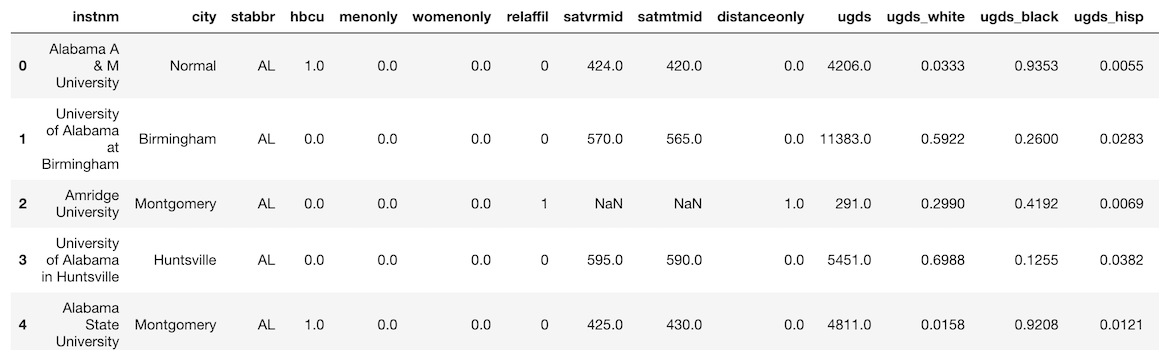``````>>> college2 = pd.read_table('data/college.csv', delimiter=',')
>>> college.equals(college2)
True
``````

## `read_table` is getting deprecated

I made a post in the Pandas Github repo suggesting that a few functions and methods that I’d like to see deprecated. The `read_table` function is getting deprecated and should never be used.

# `isna` vs `isnull` and `notna` vs `notnull`

The `isna` and `isnull` methods both determine whether each value in the DataFrame is missing or not. The result will always be a DataFrame (or Series) of all boolean values.

These methods are exactly the same. We say that one is an alias of the other. There is no need for both of them in the library. The `isna` method was added more recently because the characters `na` are found in other missing value methods such as `dropna` and `fillna`. Confusingly, Pandas uses `NaN`, `None`, and `NaT` as missing value representations and not `NA`.

`notna` and `notnull` are aliases of each other as well and simply return the opposite of `isna`. There’s no need for both of them.

Let’s verify that `isna` and `isnull` are aliases.

``````>>> college_isna = college.isna()
>>> college_isnull = college.isnull()
>>> college_isna.equals(college_isnull)
True
``````

## I only use `isna` and `notna`

I use the methods that end in `na` to match the names of the other missing value methods `dropna` and `fillna`.

You can also avoid ever using `notna` since Pandas provides the inversion operator, `~` to invert boolean DataFrames.

# Arithmetic and Comparison Operators and their Corresponding Methods

All arithmetic operators have corresponding methods that function similarly.

• `+` - `add`
• `-` - `sub` and `subtract`
• `*` - `mul` and `multiply`
• `/` - `div`, `divide` and `truediv`
• `**` - `pow`
• `//` - `floordiv`
• `%` - `mod`

All the comparison operators also have corresponding methods.

• `>` - `gt`
• `<` - `lt`
• `>=` - `ge`
• `<=` - `le`
• `==` - `eq`
• `!=` - `ne`

Let’s select the undergraduate population column, `ugds` as a Series, add 100 to it and verify that both the plus operator its corresponding method, `add`, give the same result.

``````>>> ugds = college['ugds']
>>> ugds_operator = ugds + 100
>>> ugds_operator.equals(ugds_method)
True
``````

## Calculating the z-scores of each school

Let’s do a slightly more complex example. Below, we set the index to be the institution name and then select both of the SAT columns. We remove schools that do not provide these scores with `dropna`.

``````>>> college_idx = college.set_index('instnm')
>>> sats = college_idx[['satmtmid', 'satvrmid']].dropna()
``````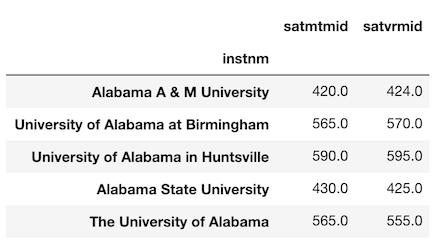Let’s say we are interested in finding the z-score for each college’s SAT score. To calculate this, we would need to subtract the mean and divide by the standard deviation. Let’s first calculate the mean and standard deviation of each column.

``````>>> mean = sats.mean()
>>> mean
satmtmid    530.958615
satvrmid    522.775338
dtype: float64
>>> std = sats.std()
>>> std
satmtmid    73.645153
satvrmid    68.591051
dtype: float64
``````

Let’s now use the arithmetic operators to complete the calculation.

``````>>> zscore_operator = (sats - mean) / std
``````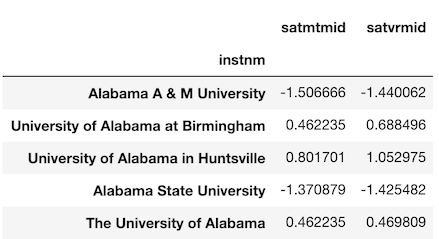Let’s repeat this with their corresponding methods and verify equality.

``````>>> zscore_methods = sats.sub(mean).div(std)
>>> zscore_operator.equals(zscore_methods)
True
``````

If you are enjoying this article, consider purchasing the All Access Pass! which includes all my current and future material for one low price.

## An actual need for the method

So far we haven’t seen an explicit need for the methods over the operators. Let’s see an example where we absolutely need the method to complete the task. The college dataset contains 9 consecutive columns holding the relative frequency of the undergraduate population by race. The first column is `ugds_white` and the last `ugds_unkn`. Let’s select these columns now into their own DataFrame.

``````>>> college_race = college_idx.loc[:, ‘ugds_white’:’ugds_unkn’]
``````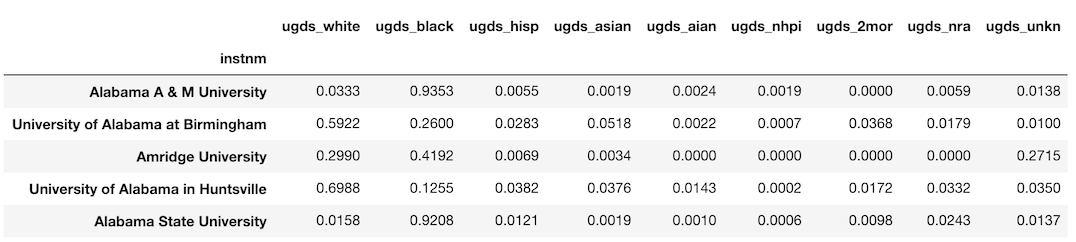Let’s say we are interested in the raw count of the student population by race per school. We need to multiply the total undergraduate population by each column. Let’s select the `ugds` column as a Series.

``````>>> ugds = college_idx['ugds']
instnm
Alabama A & M University                4206.0
University of Alabama at Birmingham    11383.0
Amridge University                       291.0
University of Alabama in Huntsville     5451.0
Alabama State University                4811.0
Name: ugds, dtype: float64
``````

We then multiply the `college_race` DataFrame by this Series. Intuitively, this seems like it should work, but it does not. Instead, it returns an enormous DataFrame with 7,544 columns.

``````>>> df_attempt = college_race * ugds
``````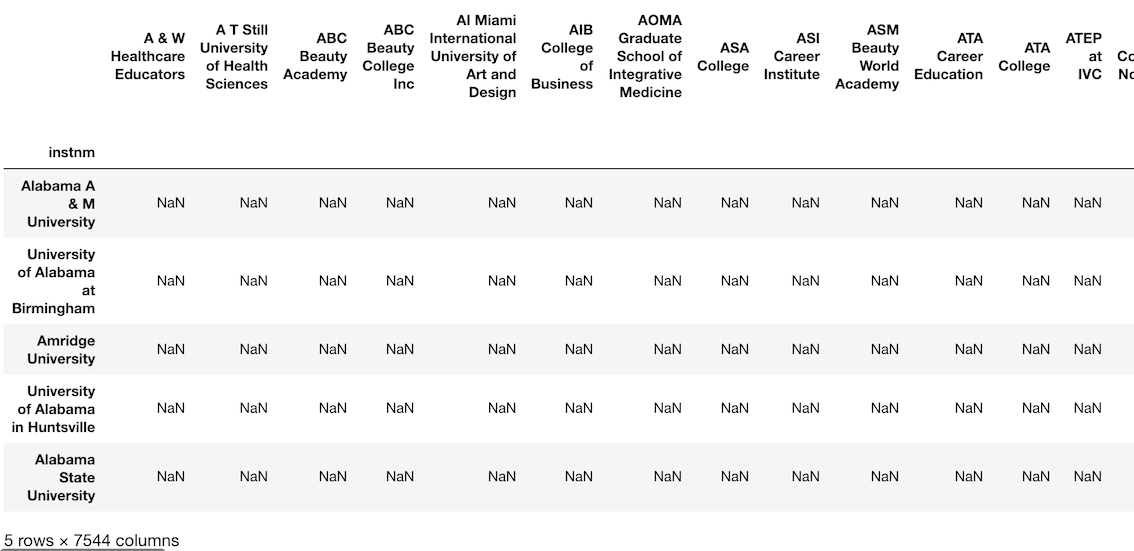``````>>> df_attempt.shape
(7535, 7544)
``````

## Automatic alignment on the index and/or columns

Whenever an operation happens between two Pandas objects, an alignment always takes place between the index and/or columns of the two objects. In the above operation, we multiplied the `college_race` DataFrame and the `ugds` Series together. Pandas automatically (implicitly) aligned the columns of `college_race` to the index values of `ugds`.

None of the `college_race` columns match the index values of `ugds`. Pandas does the alignment by performing an outer join keeping all values that match as well as those that do not. This returns a ridiculous looking DataFrame with all missing values. Scroll all the way to the right to view the original column names of the `college_race` DataFrame.

## Change the direction of the alignment with a method

All operators only work in a single way. We cannot change how the multiplication operator, `*`, works. Methods, on the other hand, can have parameters that we can use to control how the operation takes place.

## Use the `axis` parameter of the `mul` method

All the methods that correspond to the operators listed above have an `axis` parameter that allows us to change the direction of the alignment. Instead of aligning the columns of a DataFrame to the index of a Series, we can align the index of a DataFrame to the index of a Series. Let’s do that now so that we can find the answer to our problem from above.

``````>>> df_correct = college_race.mul(ugds, axis='index').round(0)
``````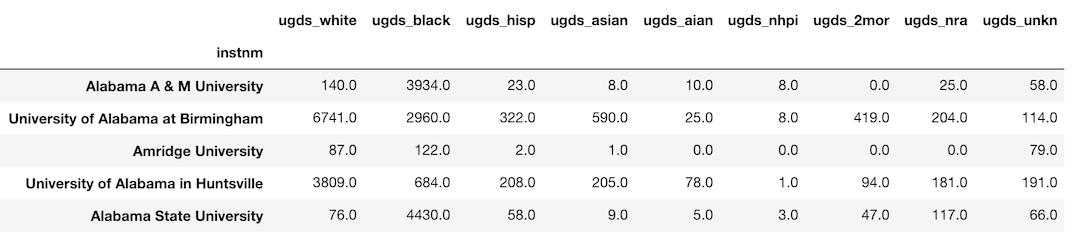By default, the `axis` parameter is set to ‘columns’. We changed it to ‘index’ so that a proper alignment took place

## Guidance: Only use the arithmetic and comparison methods when absolutely necessary, otherwise use the operators

The arithmetic and comparison operators are more common and should be attempted first. If you come across a case where the operator does not complete the task, then use the method.

# Builtin Python functions vs Pandas methods with the same name

There are a few DataFrame/Series methods that return the same result as a builtin Python function with the same name. They are:

• `sum`
• `min`
• `max`
• `abs`

Let’s verify that they give the same result by testing them out on a single column of data. We begin by selecting the non-missing values of the undergraduate student population column, `ugds`.

``````>>> ugds = college['ugds'].dropna()
0     4206.0
1    11383.0
2      291.0
3     5451.0
4     4811.0
Name: ugds, dtype: float64
``````

## Verifying sum

``````>>> sum(ugds)
16200904.0
>>> ugds.sum()
16200904.0
``````

## Verifying max

``````>>> max(ugds)
151558.0
>>> ugds.max()
151558.0
``````

## Verifying min

``````>>> min(ugds)
0.0
>>> ugds.min()
0.0
``````

## Verifying abs

``````>>> abs(ugds).head()
0     4206.0
1    11383.0
2      291.0
3     5451.0
4     4811.0
Name: ugds, dtype: float64
0     4206.0
1    11383.0
2      291.0
3     5451.0
4     4811.0
Name: ugds, dtype: float64
``````

## Time the performance of each

Let’s see if there is a performance difference between each method.

## sum performance

``````>>> %timeit sum(ugds)
644 µs ± 80.3 µs per loop
>>> %timeit -n 5 ugds.sum()
164 µs ± 81 µs per loop
``````

## max performance

``````>>> %timeit -n 5 max(ugds)
717 µs ± 46.5 µs per loop
>>> %timeit -n 5 ugds.max()
172 µs ± 81.9 µs per loop
``````

## min performance

``````>>> %timeit -n 5 min(ugds)
705 µs ± 33.6 µs per loop
>>> %timeit -n 5 ugds.min()
151 µs ± 64 µs per loop
``````

## abs performance

``````>>> %timeit -n 5 abs(ugds)
138 µs ± 32.6 µs per loop
>>> %timeit -n 5 ugds.abs()
128 µs ± 12.2 µs per loop
``````

## Performance discrepancy for `sum`, `max`, and `min`

There are clear performance discrepancies for `sum`, `max`, and `min`. Completely different code is executed when these builtin Python functions are used as opposed to when the Pandas method is called. Calling `sum(ugds)` essentially creates a Python for loop to iterate through each value one at a time. On the other hand, calling `ugds.sum()` executes the internal Pandas `sum` method which is written in C and much faster than iterating with a Python for loop.

There is a lot of overhead in Pandas which is why the difference is not greater. If we instead create a NumPy array and redo the timings, we can see an enormous difference with the Numpy array `sum` outperforming the Python `sum` function by a factor of 200 on an array of 10,000 floats.

## No Performance difference for `abs`

Notice that there is no performance difference when calling the `abs` function versus the `abs` Pandas method. This is because the exact same underlying code is being called. This is due to how Python chose to design the `abs` function. It allows developers to provide a custom method to be executed whenever the `abs` function is called. Thus, when you write `abs(ugds)`, you are really calling `ugds.abs()`. They are literally the same.

# Standardizing `groupby Aggregation`

There are a number of syntaxes that get used for the `groupby` method when performing an aggregation. I suggest choosing a single syntax so that all of your code looks the same.

## The three components of `groupby aggregation`

Typically, when calling the `groupby` method, you will be performing an aggregation. This is the by far the most common scenario. When you are performing an aggregation during a `groupby`, there will always be three components.

• Grouping column — Unique values form independent groups
• Aggregating column — Column whose values will get aggregated. Usually numeric
• Aggregating function — How the values will get aggregated (sum, min, max, mean, median, etc…)

## My syntax of choice for `groupby`

There are a few different syntaxes that Pandas allows to perform a groupby aggregation. The following is the one I use.

`df.groupby('grouping column').agg({'aggregating column': 'aggregating function'})`

## A buffet of `groupby` syntaxes for finding the maximum math SAT score per state

Below, we will cover several different syntaxes that return the same (or similar) result for finding the maximum SAT score per state. Let’s look at the data we will be using first.

``````>>> college[['stabbr', 'satmtmid', 'satvrmid', 'ugds']].head()
``````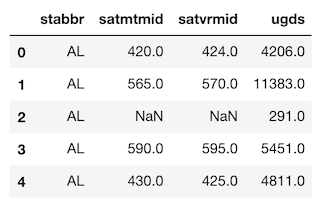Method 1: Here is my preferred way of doing the groupby aggregation. It handles complex cases.

``````>>> college.groupby('stabbr').agg({'satmtmid': 'max'}).head()

``````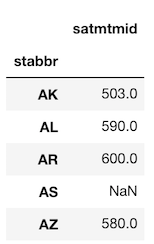Method 2a: The aggregating column can be selected within brackets following the call to `groupby`. Notice that a Series is returned here and not a DataFrame.

``````>>> college.groupby('stabbr')['satmtmid'].agg('max').head()
stabbr
AK    503.0
AL    590.0
AR    600.0
AS      NaN
AZ    580.0
Name: satmtmid, dtype: float64
``````

Method 2b: The `aggregate` method is an alias for `agg` and can also be used. This returns the same Series as above.

``````>>> college.groupby('stabbr')['satmtmid'].aggregate('max').head()
``````

Method 3: You can call the aggregating method directly without calling `agg`. This returns the same Series as above.

``````>>> college.groupby('stabbr')['satmtmid'].max().head()
``````

## Major benefits of preferred syntax

The reason I choose this syntax is that it can handle more complex grouping problems. For instance, if we wanted to find the max and min of the math and verbal sat scores along with the average undergrad population per state we would do the following.

``````>>> df.groupby('stabbr').agg({'satmtmid': ['min', 'max'],
'satvrmid': ['min', 'max'],
``````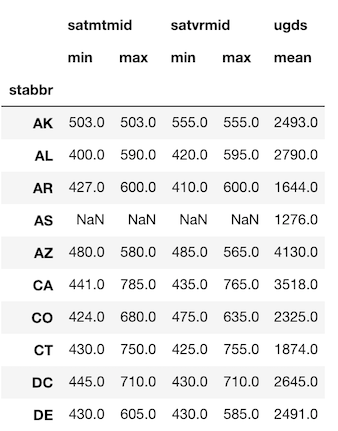This problem isn’t solvable using the other syntaxes.

# Handling a MultiIndex

A MultiIndex or multi-level index is a cumbersome addition to a Pandas DataFrame that occasionally makes data easier to view, but often makes it more difficult to manipulate. You usually encounter a MultiIndex after a call to`groupby` when using multiple grouping columns or multiple aggregating columns.

Let’s create a result similar to the last groupby from above, except this time group by both state and religious affiliation.

``````>>> agg_dict = {'satmtmid': ['min', 'max'],
'satvrmid': ['min', 'max'],
'ugds': 'mean'}
>>> df = college.groupby(['stabbr', 'relaffil']).agg(agg_dict)
``````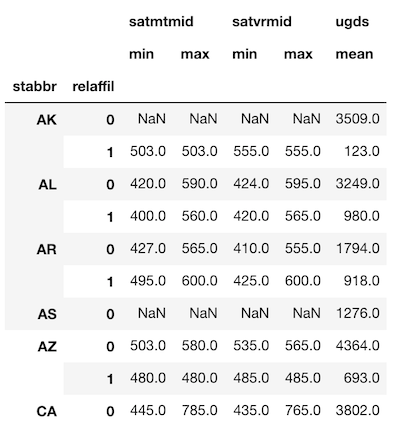## A MultiIndex in both the index and columns

Both the rows and columns have a MultiIndex with two levels.

## Selection and further processing is difficult with a MultiIndex

There is little extra functionality that a MultiIndex adds to your DataFrame. They have different syntax for making subset selections and are more difficult to use with other methods. If you are an expert Pandas user, you can get some performance gains when making subset selections, though I typically do not like the added complexity that they come with. I suggest working with DataFrames that have a simpler, single-level index.

## Convert to a single level index — Rename the columns and reset the index

We can convert this DataFrame so that only single-level indexes remain. There is no direct way to rename columns of a DataFrame during a groupby (yes, something so simple is impossible with pandas), so we must overwrite them manually. Let’s do that now.

``````>>>> df.columns = ['min satmtmid', 'max satmtmid', 'min satvrmid',
'max satvrmid', 'mean ugds']
``````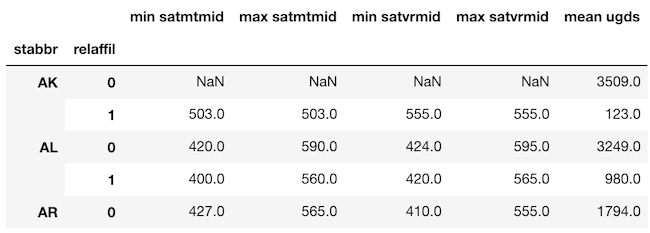From here, we can use the `reset_index` method to make each index level an actual column.

``````>>> df.reset_index().head()
``````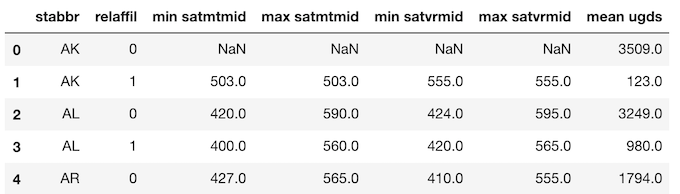# The similarity between groupby, pivot_table, and crosstab

Some users might be surprised to find that a`groupby` (when aggregating), `pivot_table`, and `pd.crosstab` are essentially identical. However, there are specific use cases for each, so all still meet the threshold for being included in a minimally sufficient subset of Pandas.

## The equivalency of `groupby` aggregation and `pivot_table`

Performing an aggregation with `groupby` is essentially equivalent to using the `pivot_table` method. Both methods return the exact same data, but in a different shape. Let’s see a simple example that proves that this is the case. We will use a new dataset containing employee demographic information from the city of Houston.

``````>>> emp = pd.read_csv('data/employee.csv')
``````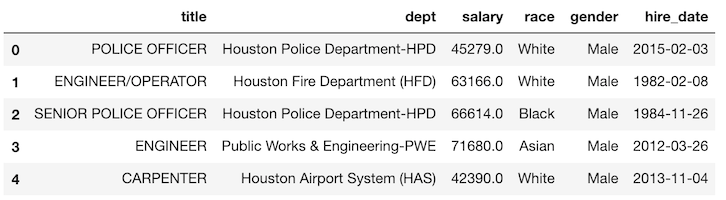Let’s use a `groupby` to find the average salary for each department by gender.

``````>>> emp.groupby(['dept', 'gender']).agg({'salary':'mean'}).round(-3)

``````We can duplicate this data by using a `pivot_table`.

``````>>> emp.pivot_table(index='dept', columns='gender',
values='salary', aggfunc='mean').round(-3)
``````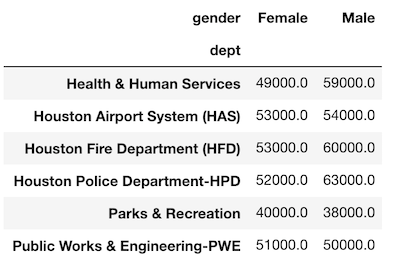Notice that the values are exactly the same. The only difference is that the gender column has been pivoted so its unique values are now the column names. The same three components of a `groupby` are found in a `pivot_table`. The grouping column(s) are passed to the `index` and `columns` parameters. The aggregating column is passed to the `values` parameter and the aggregating function is passed to the `aggfunc` parameter.

It’s actually possible to get an exact duplication of both the data and the shape by passing both grouping columns as a list to the `index` parameter.

``````>>> emp.pivot_table(index=['dept','gender'],
values='salary', aggfunc='mean').round(-3)
``````

Typically, `pivot_table` is used with two grouping columns, one as the `index` and the other as the `columns`. But, it can be used for a single grouping column. The following produces an exact duplication of a single grouping column with `groupby`.

``````>>> df1 = emp.groupby('dept').agg({'salary':'mean'}).round(0)
>>> df2 = emp.pivot_table(index='dept', values='salary',
aggfunc='mean').round(0)
>>> df1.equals(df2)
True
``````

## Guidance: use `pivot_table` when comparing groups

I really like to use pivot tables to compare values across groups and a `groupby` when I want to continue an analysis. From above, it is easier to compare male to female salaries when using the output of `pivot_table`. The result is easier to digest as a human and its the type of data you will see in an article or blog post. I view pivot tables as a finished product.

The result of a `groupby` is going to be in tidy form, which lends itself to easier subsequent analysis, but isn’t as interpretable.

## The equivalency of pivot_table and pd.crosstab

The `pivot_table` method and the `crosstab` function can both produce the exact same results with the same shape. They both share the parameters `index`, `columns`, `values`, and `aggfunc`. The major difference on the surface is that `crosstab` is a function and not a DataFrame method. This forces you to use columns as Series and not string names for the parameters. Let’s see an example taking the average salary by gender and race.

``````>>> emp.pivot_table(index='gender', columns='race',
values='salary', aggfunc='mean').round(-3)
``````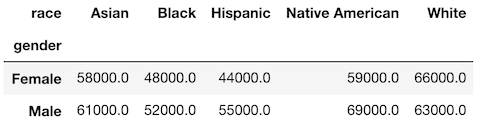The `crosstab` function produces the exact same result with the following syntax.

``````>>> pd.crosstab(index=emp['gender'], columns=emp['race'],
values=emp['salary'], aggfunc='mean').round(-3)
``````

## crosstab was built for counting

A crosstabulation (also known as a contingency table) shows the frequency between two variables. This is the default functionality for `crosstab` if given two columns. Let’s show this by counting the frequency of all race and gender combinations. Notice that there is no need to provide an `aggfunc`.

``````>>> pd.crosstab(index=emp['gender'], columns=emp['race'])
``````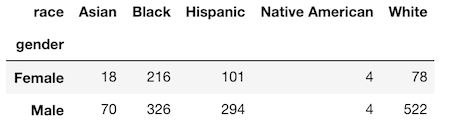The `pivot_table` method can duplicate this but you must use the `size` aggregation function.

``````>>> emp.pivot_table(index='gender', columns='race', aggfunc='size')
``````

## Relative frequency — the unique functionality with crosstab

At this point, it appears that the `crosstab` function is just a subset of `pivot_table`. But, there is a single unique functionality that it posseses that makes it potentially worthwhile to add to your minimally sufficient subset. It has the ability to calculate relative frequencies across groups with the `normalize` parameter. For instance, if we wanted the percentage breakdown by gender across each race we can set the `normalize` parameter to ‘columns’.

``````>>> pd.crosstab(index=emp['gender'], columns=emp['race'],
normalize='columns').round(2)
``````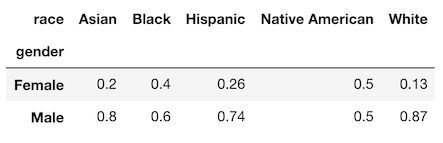You also have the option of normalizing over the rows using the string ‘index’ or over the entire DataFrame with the string ‘all’ as seen below.

``````>>> pd.crosstab(index=emp['gender'], columns=emp['race'],
normalize='all').round(3)
``````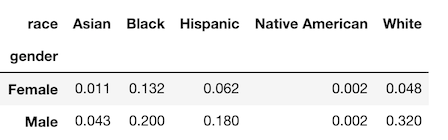## Guidance: Only use crosstab when finding relative frequency

All other situations where the `crosstab` function may be used can be handled with `pivot_table`. It is possible to manually calculate the relative frequencies after running `pivot_table` so `crosstab` isn’t all that necessary. But, it does do this calculation in a single readable line of code, so I will continue to use it.

# pivot vs pivot_table

There exists a `pivot` method that is nearly useless and can basically be ignored. It functions similarly to `pivot_table` but does not do any aggregation. It only has three parameters, `index`, `columns`, and `values`. All three of these parameters are present in `pivot_table`. It reshapes the data without an aggregation. Let’s see an example with a new simple dataset.

``````>>> df = pd.read_csv('data/state_fruit.csv')
>>> df
``````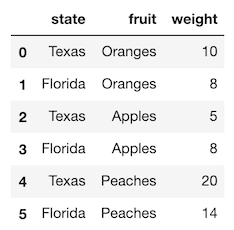Let’s use the `pivot` method to reshape this data so that the fruit names become the columns and the weight becomes the values.

``````>>> df.pivot(index='state', columns='fruit', values='weight')
``````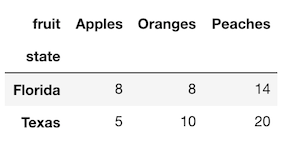Using the `pivot` method, reshapes the data without aggregating or doing anything to it. `pivot_table`, on the other hand, requires that you do an aggregation. In this case, there is only one value per intersection of state and fruit, so many aggregation functions will return the same value. Let’s recreate this exact same table with the max aggregation function.

``````>>> df.pivot_table(index='state', columns='fruit',
values='weight', aggfunc='max')
``````

## Issues with pivot

There are a couple major issues with the `pivot` method. First, it can only handle the case when both `index` and `columns` are set to a single column. If you want to keep multiple columns in the index then you cannot use `pivot`. Also, if any combination of `index` and `columns` appear more than once, then you will get an error as it does not perform an aggregation. Let’s produce this particular error with a dataset that is similar to the above but adds two additional rows.

``````>>> df2 = pd.read_csv('data/state_fruit2.csv')
>>> df2
``````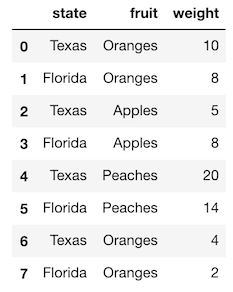Attempting to pivot this will not work as now the combination for both Texas and Florida with Oranges have multiple rows.

``````>>> df2.pivot(index='state', columns='fruit', values='weight')
ValueError: Index contains duplicate entries, cannot reshape
``````

If you would like to reshape this data, you will need to decide on how you would like to aggregate the values.

## Guidance — Consider using only `pivot_table and not pivot`

`pivot_table` can accomplish all of what `pivot` can do. In the case that you do not need to perform an aggregation, you still must provide an aggregation function.

# The similarity between melt and stack

The `melt` and `stack` methods reshape data in the same exact manner. The major difference is that the `melt` method does not work with data in the index while `stack` does. It’s easier to describe how they work with an example. Let’s begin by reading in a small dataset of arrival delay of airlines for a few airports.

``````>>> ad = pd.read_csv('data/airline_delay.csv')
``````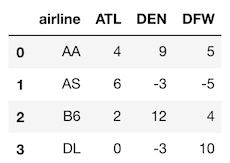Let’s reshape this data so that we have three columns, the airline, the airport and the arrival delay. We will begin with the `melt` method, which has two main parameters, `id_vars` which are the column names that are to remain vertical (and not reshaped) and `value_vars` which are the column names to be reshaped into a single column.

``````>>> ad.melt(id_vars='airline', value_vars=['ATL', 'DEN', 'DFW'])
``````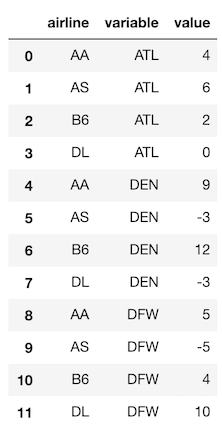The `stack` method can produce nearly identical data, but it places the reshaped column in the index. It also preserves the current index. To recreate the data above, we need to set the index to the column(s) that will not be reshaped first. Let’s do that now.

``````>>> ad_idx = ad.set_index('airline')
``````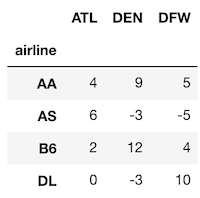Now, we can use `stack` without setting any parameters to get nearly the same result as `melt`.

``````>>> ad_idx.stack()
airline
AA       ATL     4
DEN     9
DFW     5
AS       ATL     6
DEN    -3
DFW    -5
B6       ATL     2
DEN    12
DFW     4
DL       ATL     0
DEN    -3
DFW    10
dtype: int64
``````

This returns a Series with a MultiIndex with two levels. The data values are the same, but in a different order. Calling `reset_index` will get us back to a single-index DataFrame.

``````>>> ad_idx.stack().reset_index()
``````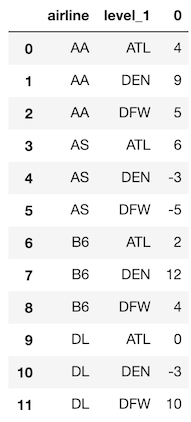## Renaming columns with `melt`

I prefer `melt` as you can rename columns directly and you can avoid dealing with a MultiIndex. The `var_name` and `value_name` parameters are provided to `melt` to rename the reshaped columns. It’s also unnecessary to list out all of the columns you are melting because all the columns not found in `id_vars` will be reshaped.

``````>>> ad.melt(id_vars='airline', var_name='airport',
value_name='arrival delay')
``````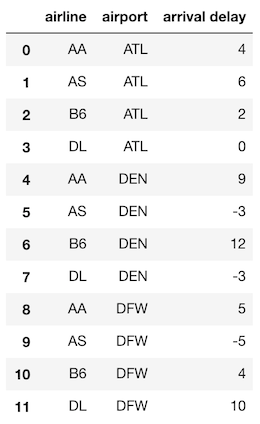# The Similarity between pivot and unstack

We’ve already seen how the `pivot` method words. `unstack` is its analog that works with values in the index. Let’s look at the simple DataFrame that we used with `pivot`.

``````>>> df = pd.read_csv('data/state_fruit.csv')
>>> df
``````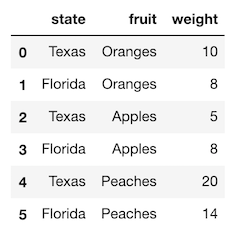The `unstack` method pivots values in the index. We must set the index to contain the columns that we would have used as the `index` and `columns` parameters in the `pivot` method. Let’s do that now.

``````>>> df_idx = df.set_index(['state', 'fruit'])
>>> df_idx
``````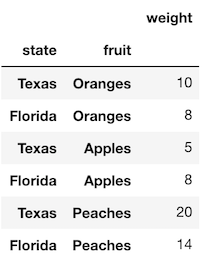Now we can use `unstack` without any parameters, which will pivot the index level closest to the actual data (the fruit column) so that its unique values become the new column names.

``````>>> df_idx.unstack()
``````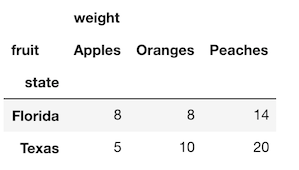The result is nearly identical to what was returned with the `pivot` method except now we have a MultiIndex for the columns.

## Guidance — Use `pivot_table` over `unstack` or `pivot`

Both `pivot` and `unstack` work similarly but from above, `pivot_table` can handle all cases that `pivot` can, so I suggest using it over both of the others.

# End of Specific Examples

The above specific examples cover many of the most common tasks within Pandas where there are multiple different approaches you can take. For each example, I argued for using a single approach. This is the approach that I use when doing a data analysis with Pandas and the approach I teach to my students.

# The Zen of Python

Minimally Sufficient Python was inspired by the Zen of Python, a list of 19 aphorisms giving guidance for language usage by Tim Peters. The aphorism in particular worth noting is the following:

There should be one-- and preferably only one --obvious way to do it.

I find that the Pandas library disobeys this guidance more than any other library I have encountered. Minimally Sufficient Pandas is an attempt to steer users so that this principle is upheld.

## Pandas Style Guide

While the specific examples above provide guidance for many tasks, it is not an exhaustive list that covers all corners of the library. You may also disagree with some of the guidance.

To help you use the library I recommend creating a “Pandas style guide”. This isn’t much different than coding style guides that are often created so that codebases look similar. This is something that greatly benefits teams of analysts that all use Pandas. Enforcing a Pandas style guide can help by:

• Having all common data analysis tasks use the same syntax
• Making it easier to put Pandas code in production
• Reducing the chance of landing on a Pandas bug. There are thousands of open issues. Using a smaller subset of the library will help avoid these.

# Best of the API

The Pandas DataFrame API is enormous. There are dozens of methods that have little to no use or are aliases. Below is my list of all the DataFrame attributes and methods that I consider sufficient to complete nearly any task.

• columns
• dtypes
• index
• shape
• T
• values

• all
• any
• count
• describe
• idxmax
• idxmin
• max
• mean
• median
• min
• mode
• nunique
• sum
• std
• var

• abs
• clip
• corr
• cov
• cummax
• cummin
• cumprod
• cumsum
• diff
• nlargest
• nsmallest
• pct_change
• prod
• quantile
• rank
• round

• iloc
• loc
• tail

• dropna
• fillna
• interpolate
• isna
• notna

• expanding
• groupby
• pivot_table
• resample
• rolling

• append
• merge

## Other

• asfreq
• astype
• copy
• drop
• drop_duplicates
• equals
• isin
• melt
• plot
• rename
• replace
• reset_index
• sample
• select_dtypes
• shift
• sort_index
• sort_values
• to_csv
• to_json
• to_sql

• pd.concat
• pd.crosstab
• pd.cut
• pd.qcut